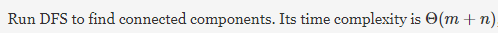# Gate CS-2008 Question Paper With Solutions

Q. 1 The most efficient algorithm for finding the number of connected components in
an undirected graph on n vertices and m edges has time complexity.

(A) Q(n)

(B) Q(m)

(C) Q(m + n)

(D) Q(mn)